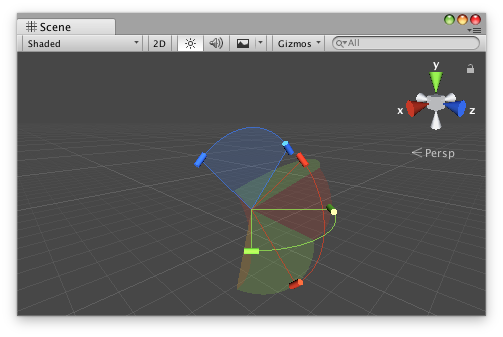Version: 2018.4
LanguageEnglish
• C#

# JointAngularLimitHandle

class in UnityEditor.IMGUI.Controls

/

Implemented in:UnityEditor

Suggest a change

## Success!

Thank you for helping us improve the quality of Unity Documentation. Although we cannot accept all submissions, we do read each suggested change from our users and will make updates where applicable.

Close

## Submission failed

For some reason your suggested change could not be submitted. Please <a>try again</a> in a few minutes. And thank you for taking the time to help us improve the quality of Unity Documentation.

Close

## Description

A class for a compound handle to edit multiaxial angular motion limits in the Scene view.JointAngularLimitHandle in the Scene View.

The shapes rendered by the DrawHandle method assume that angular limits are applied first along the x-axis, then the y-axis, and finally the z-axis.

The following component defines angular limits for a CharacterJoint to be added at run time.

```using UnityEngine;public class JointExample : MonoBehaviour
{
public float xMin { get { return m_XMin; } set { m_XMin = value; } }
[SerializeField]
float m_XMin = -45f;    public float xMax { get { return m_XMax; } set { m_XMax = value; } }
[SerializeField]
float m_XMax = 45f;    public float yMax { get { return m_YMax; } set { m_YMax = value; } }
[SerializeField]
float m_YMax = 45f;    public float zMax { get { return m_ZMax; } set { m_ZMax = value; } }
[SerializeField]
float m_ZMax = 45f;    protected virtual void Start()
{
var joint = gameObject.AddComponent<CharacterJoint>();        var limit = joint.lowTwistLimit;
limit.limit = m_XMin;
joint.lowTwistLimit = limit;        limit = joint.highTwistLimit;
limit.limit = m_XMax;
joint.highTwistLimit = limit;        limit = joint.swing1Limit;
limit.limit = m_YMax;
joint.swing1Limit = limit;        limit = joint.swing2Limit;
limit.limit = m_ZMax;
joint.swing2Limit = limit;
}
}
```

The following Custom Editor example allows you to edit the serialized angular limits in the Scene view.

```using UnityEditor;
using UnityEditor.IMGUI.Controls;
using UnityEngine;[CustomEditor(typeof(JointExample)), CanEditMultipleObjects]
public class JointExampleEditor : Editor
{
JointAngularLimitHandle m_Handle = new JointAngularLimitHandle();    // the OnSceneGUI callback uses the Scene view camera for drawing handles by default
protected virtual void OnSceneGUI()
{
var jointExample = (JointExample)target;        // copy the target object's data to the handle
m_Handle.xMin = jointExample.xMin;
m_Handle.xMax = jointExample.xMax;        // CharacterJoint and ConfigurableJoint implement y- and z-axes symmetrically
m_Handle.yMin = -jointExample.yMax;
m_Handle.yMax = jointExample.yMax;        m_Handle.zMin = -jointExample.zMax;
m_Handle.zMax = jointExample.zMax;        // set the handle matrix to match the object's position/rotation with a uniform scale
Matrix4x4 handleMatrix = Matrix4x4.TRS(
jointExample.transform.position,
jointExample.transform.rotation,
Vector3.one
);        EditorGUI.BeginChangeCheck();        using (new Handles.DrawingScope(handleMatrix))
{
// maintain a constant screen-space size for the handle's radius based on the origin of the handle matrix
m_Handle.radius = HandleUtility.GetHandleSize(Vector3.zero);            // draw the handle
EditorGUI.BeginChangeCheck();
m_Handle.DrawHandle();
if (EditorGUI.EndChangeCheck())
{
// record the target object before setting new values so changes can be undone/redone
Undo.RecordObject(jointExample, "Change Joint Example Properties");                // copy the handle's updated data back to the target object
jointExample.xMin = m_Handle.xMin;
jointExample.xMax = m_Handle.xMax;                jointExample.yMax = m_Handle.yMax == jointExample.yMax ? -m_Handle.yMin : m_Handle.yMax;                jointExample.zMax = m_Handle.zMax == jointExample.zMax ? -m_Handle.zMin : m_Handle.zMax;
}
}
}
}
```